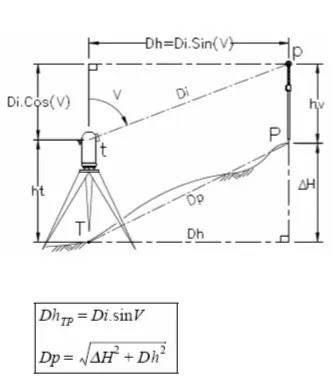surveying indirect leveling ₪ 1.1 Principe

# surveying indirect leveling ₪ 1.1 Principe

Trigonometric indirect topography meveming make it possible to determine the height difference ΔH entre la station T d’un théodolite et un point P. This is done by measuring the inclined distance alog the line of sight Di and zenith angle denoted in the figure opposite. From the diagram, we can write that:ΔHTP is the height difference from T to P
ht is the station height (or trunnion height).
hv is the height of the indicator or more generally the height targeted above the point sought (you can also put a surveying staff at P).
We deduce the horizontal distance Dh
We deduce the distance according to the slope Dp :
There are 2 cases which depend on whether or not it is possible to measure the distance Dp.